An Introduction to Interferometers for Highly Accurate Engineering Measurements
Dr Jody Muelaner posted on September 29, 2020 |
How interferometers work, what affects their accuracy, and how they are used in manufacturing.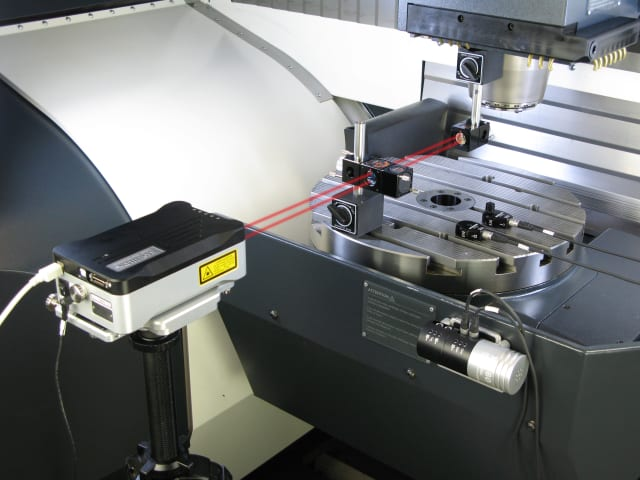Interferometers use the interfere between waves, such as light, radio or sound, to make a measurement. (Image courtesy of Renishaw.)

In its broadest sense, an interferometer is any instrument that uses the interfere between waves, such as light, radio or sound, to make a measurement. Within engineering, interferometry almost always refers to interference between light, generally lasers. These laser interferometers are commonly used to measure distances, refractive index changes and surface properties. This article looks at how interferometers work, the factors that can affect their accuracy and some common engineering applications.

### How Interferometers Work

Before explaining what an interferometer is, let’s start by looking at what isn’t an interferometer. All laser measurements are not interferometry. Laser levels and straight edges simply project a laser to provide a visual reference. They use the fact that lasers are very straight and can project a line with high contrast in ambient light. This is not interferometry. It is also possible to use a laser to measure a distance without using interferometry. Low-cost laser distance measurement uses time-of-flight measurement. This involves turning a laser off and on very rapidly and observing how long it takes for the laser dot to appear. Since velocity is distance divided by time, and the speed of light is a known constant, the distance is the speed of light multiplied by the time. Time-of-flight measurement is limited in accuracy by the ability of a clock to accurately measure a very small period of time, with resolutions of typically about a millimeter. This is also not interferometry.

The oldest and simplest type of interferometer is a fringe counting Michelson-Morley experiment. Although its original form used incoherent white light, it is now usually realized using a laser. Laser light is emitted from a source and passes through a half-silvered mirror acting as a beam splitter. One beam reflects off a reference mirror while the other reflects off a measurement mirror in a spherically mounted retroreflector (SMR). The two beams are recombined at the beam splitter and directed toward a detector.(Image courtesy of the author.)

Since the two beams have traveled different distances, there is likely to be a difference in phase. If the difference in distance is an exact multiple of the wavelength of the light, then the two beams will be in phase and interference between the waves will be constructive. If the distance differs by half a wavelength, the interference will be destructive. As the measurement mirror moves, the two beams will move in and out of phase, resulting in pulses of light separated by darkness known as fringes. By counting these fringes, the displacement can be calculated in terms of the wavelength of the light.(Image courtesy of the author.)

Fringe counting interferometers can achieve extremely accurate measurements with direct traceability to primary length standards. However, they don’t directly measure distance. Instead, they measure displacement. In order to measure a distance, the displacement between the two end points must be measured, counting fringes continuously without breaking the laser beam, something that can be difficult and time-consuming in a production environment.

It is also possible to use interferometry for absolute distance measurement (ADM). This means that the distance to the measurement mirror can be directly determined without continuously counting fringes between a starting and finishing position. The laser bean can, therefore, be broken and picked up again during a displacement measurement. Not all laser ADM measurements use interferometry. The time-of-flight method mentioned above is one example.

A popular ADM technique for industrial measurements is phase detection of a modulated polarization plane, which is used by Leica in laser trackers. The phase of a reference signal is compared with the phase of a measurement signal. This may be considered as a form of interferometry since modulated waveforms are compared with each other, although these types of ADM are generally not referred to as interferometers. In any case, it overlaps in terms of application and performance.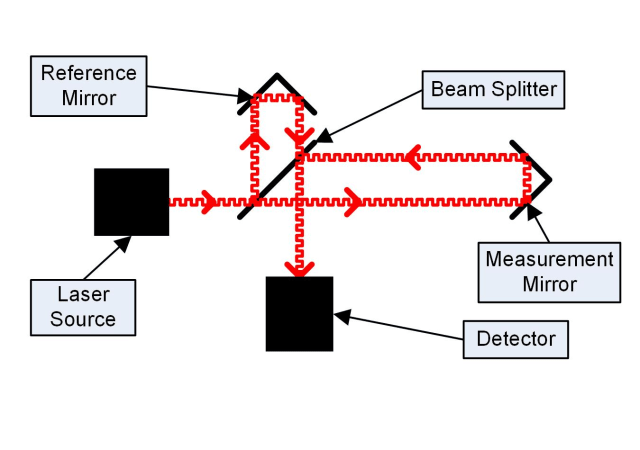(Image courtesy of the author.)

Phase detection of a modulated polarization plane uses a modulated signal rather than the waveform of the light its-self. This allows the frequency and wavelength to be easily adjusted until the reference signal and measurement signal are in-phase. The frequency f1 can be recorded before being increased to the next frequency f2 where both signals are in phase. The distance in each case is given by the following equations:d is the distance being measured.  λ1 and λ2 are the wavelengths at each recorded frequency where the signals are in-phase. N1 and N2 are the corresponding integer numbers of wavelengths over the length d.

We can use the fact that the waves were not in phase for any wavelengths between λ1 and λ2 to deduce that N2=N1+1. We can also use the fundamental wave equation to substitute the wavelength for the frequency and speed of light, c.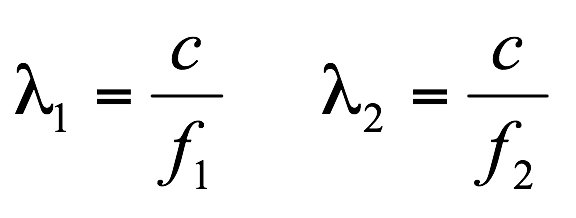Substituting and rearranging these equations gives:And finally, the distance as a function of the speed of light and the two recorded frequencies: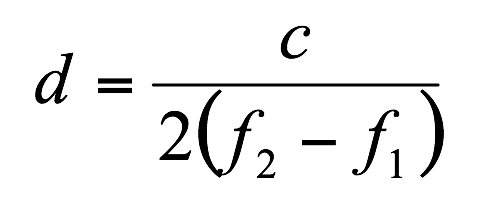ADM measurements allow beam breaks during measurement operations and make measurement in a production environment far more practical.

Another highly accurate form of ADM is known as frequency scanning interferometry (FSI). This is a similar technique but rather than adjusting the wavelength of a modulated signal, a tunable laser is used so that the wavelength of the light itself is tuned. FSI was developed by researchers at the University of Oxford to monitor the positions of detectors in the Large Hadron Collider. This required several hundred interferometers arranged in a geodetic grid so that shape changes of the particle tracker could be continuously monitored with micrometer resolution over tens of meters. This measurement system was required to maintain accuracy without any access or maintenance over a 10-year period. A particular challenge was that vibrations change the optical path distance during the laser tuning process. The FSI measurement method that emerged uses a single scanning laser source, fiber channeled to hundreds of individual interferometer lines. The measurements have direct traceability to the fundamental definition of the meter, based on the speed of light, so it needs direct calibration. It is now being adapted into industrial measurement instruments, such as the FSI based optical CMM developed by NPL and the multiline machine monitoring and calibration system developed by Etalon.

### Accuracy and Environmental Effects

Because interferometers measure distance with reference to the fundamental definition of the meter, based on the speed of light, they do not require the type of calibration that conventional instruments do. Historically, units of length measurement were defined by a physical standard, such as the Royal Cubit of ancient Egypt or the International Prototype Meter. Calibrations of physical length gauges would involve either direct comparison between the instrument and the primary standard or an unbroken series of calibrations leading back to the primary standard. Today, the meter is not defined by a physical object located at a single location. Instead, it is defined by a fundamental physical constant: the speed of light. The speed of light is defined in terms of the second. That is defined by another fundamental physical constant, the ground-state hyperfine transition frequency of the caesium 133 atom, which is the basis for atomic clocks. The calibration of instruments directly referencing fundamental standards involves calculating an uncertainty budget that combines the uncertainties in the various input quantities affecting the measurement.

Parameters affecting the uncertainty of an interferometric measurement are likely to include internal alignments, optical imperfections and the uncertainty of the frequency reference. However, unless measurements take place in a vacuum, it is typically the uncertainties that affect the refractive index of the air and dominate the uncertainty of the length measurement. The refractive index of air is affected by the temperature, pressure, humidity and CO2 concentration. The Ciddor equation can be used to determine how sensitive the refractive index is to each of these parameters. The calculation is somewhat complex but a MATLAB script is available to calculate the refractive index of air.

It is important to note that uncertainties on calibration certificates are the uncertainty of the calibration. It should not be assumed that this represents the accuracy of subsequent measurements. In fact, any subsequent measurements will include the uncertainty of the calibration plus any other random and systematic effects influencing the instrument at the time of measurement. Therefore, it is likely that the uncertainty of shop floor measurements will be significantly higher than that stated on the calibration certificate. It is common for variations in the refractive index to produce uncertainties at least an order or magnitude larger than the calibration uncertainty.

High-quality industrial laser interferometers achieve uncertainties of less than 3µm for a 10m length, a level of uncertainty that has changed little since HP released their 5526A Interferometer and 5501A Laser Transducer in 1973. These devices were intended to be integrated into machines by the machine manufacturers, providing highly accurate encoders for the control of linear axes. Using a conventional fringe counting interferometer, the uncertainty is limited by the temperature gradients in production environments. Even for a very stable environment with a temperature gradient of just 0.1°C/m, the error over a 10 m length due to refractive index changes would be 5 µm . It is, therefore, not normally possible for factory measurements to replicate the low uncertainties of measurements made in highly controlled laboratory environments.

Newer interferometers and ADM methods, including FSI, are being developed that can correct for changes in refractive index over the laser path. Some instruments already available provide some of these capabilities, such as the Etalon Laser Tracer. These methods could produce a step change in measurement capability, enabling traceable measurements with laboratory accuracy in uncontrolled factory environments.

### Applications of Interferometers

Interferometers are used for many types of industrial measurement. One common example is machine calibration. Fringe counting interferometers can be programed to measure displacement, recording a reading when the machine is stationary for a specified time period, typically around one second. The machine can then be programmed to move along an axis, stopping periodically while measurements are taken. With a simple reflector mounted on the machine axis, the position along the axis can be calibrated. Different arrangements of mirrors and prisms can be used to measure position, straightness and angular deviations such as pitch, roll and yaw. The latest machine tool calibrators use multiple lasers to measure all degrees of freedom along an axis simultaneously.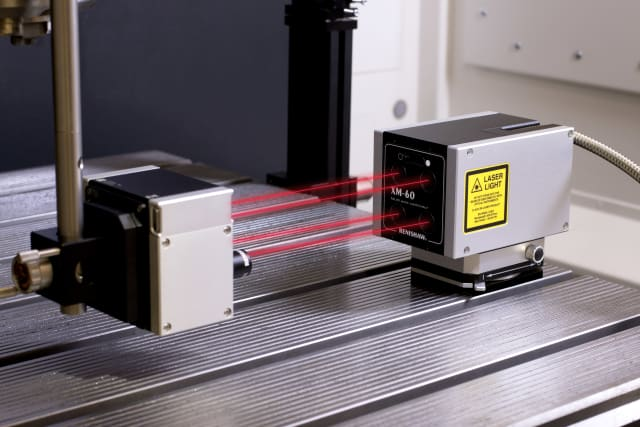Laser interferometers are commonly used to measure distances, refractive index changes and surface properties. They are often the most accurate instruments available and provide great convenience. (Image courtesy of Renishaw.)

Laser trackers are coordinate measurement instruments that can make highly accurate measurements at scales of tens of meters. They are used to set large machines and jigs and verify structures in applications such as aerospace manufacturing, power generation and shipbuilding. Laser trackers combine a laser distance measurement with two angle measurements to give coordinate measurements in three dimensions. The laser optics are mounted in a gimbal that allows the laser to be directed in over a range of angles, typically the full 360 degree range horizontally (azimuth) and a slightly more limited range vertically (elevation). The reference mirror is within the instrument, but the measurement mirror is an SMR that can be used to manually probe objects. An SMR typically contains three mirrors in a corner cube arrangement accurately aligned so that their intersection lies at the center of a steel sphere that contains them. If the laser is directed anywhere onto the face of any of the three mirrors, it will be reflected off the other mirrors and returned along a path parallel to the incoming beam. A position sensor within the laser tracker detects the offset between the outgoing and incoming beams and uses this to provide feedback to motors controlling the gimbal. In this way, the laser can be directed to the center of the SMR, at which point the returned beam overlaps and interferes with the incoming beam, enabling an interferometric distance measurement. This control happens in real-time, allowing the laser to track the SMR as it is moved around. In addition to measuring the distance to the SMR, angular encoders within the gimbal measure the azimuth and elevation angles. The coordinates of the SMR are, therefore, known with a spherical coordinate system. This can, of course, be converted into a cartesian coordinate system. In this way, the laser tracker is able to measure the coordinates to the center of the SMR. The SMR has a known calibrated radius, so it can be used as a probe with which objects can be measured.(Image courtesy of the author.)

Although a single laser tracker measurement can give the coordinates of a measured position, the uncertainties for the angles are considerably larger than the uncertainty of the distance. This results in a dish-shaped uncertainty field within which the target may be located. If this region of uncertainty is too large, it can be reduced by making additional measurements, approaching the target from angles as close to perpendicular to the original measurement as possible. Displacement measurements can then be used to measure 3D coordinates, using a technique called multilateration, without involving the angular measurements. Multilateration is similar to triangulation but instead of combining angle measurements taken from multiple positions, it uses multiple displacements between coordinates measured from multiple positions. Three distance measurements are sufficient to calculate a 3D position. If additional redundant measurements are included, then averaging can reduce refractive index distortions while residuals can provide an indication of the uncertainty.

Other applications include surface roughness, where a full field measurement can be used to observe fringes over a field of view. The fringes indicate deviations from a perfectly flat surface.

In many applications, interferometry provides the most traceable measurements with the lowest uncertainty. Further advances, especially to correct for refractive index changes, will enable even higher performance in the future.

 Recommended For You